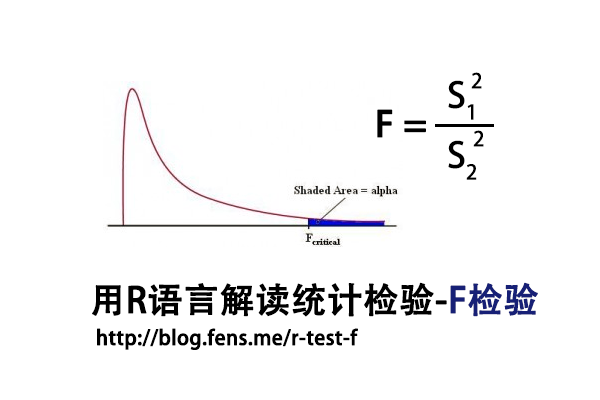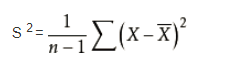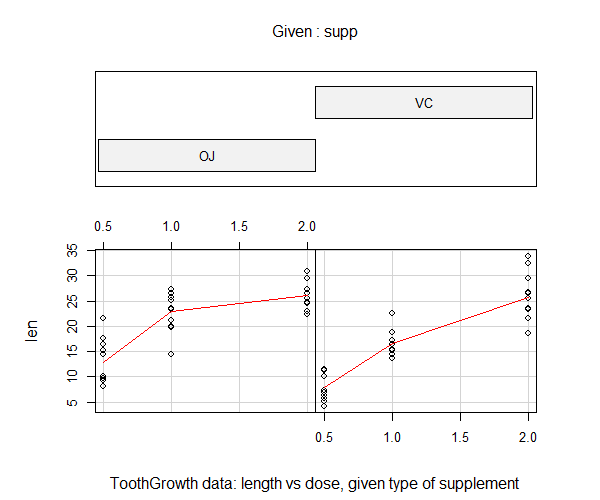• Posts tagged "F-Test"

# Blog Archives

## 用R语言解读统计检验-F检验

R的极客理想系列文章，涵盖了R的思想，使用，工具，创新等的一系列要点，以我个人的学习和体验去诠释R的强大。

R语言作为统计学一门语言，一直在小众领域闪耀着光芒。直到大数据的爆发，R语言变成了一门炙手可热的数据分析的利器。随着越来越多的工程背景的人的加入，R语言的社区在迅速扩大成长。现在已不仅仅是统计领域，教育，银行，电商，互联网….都在使用R语言。

• 张丹(Conan), 程序员/Quant: Java,R,Nodejs
• blog: http://blog.fens.me
• email: bsspirit@gmail.com

http://blog.fens.me/r-test-f1. F检验介绍
2. 数据集
3. F检验实现

## 1. F检验介绍

F检验法(F-test)，初期叫方差比率检验(Variance Ratio)，又叫联合假设检验(Joint Hypotheses Test)，是英国统计学家Fisher提出的，主要通过比较两组数据的方差，以确定他们的密度是否有显著性差异。至于两组数据之间是否存在系统误差，则在进行F检验并确定它们的密度没有显著性差异之后，再进行T检验

F检验，是一种在零假设(H0)之下，统计值服从F-分布的检验。F统计量计算公式：

• F:统计量，根据自由度查表，当F值小于查表值时没有显著差异，当F值大于等于查表值时有显著差异
• S1:样本1的标准差
• S2:样本2的标准差
• 分子自由度: df=分子的数量-1
• 分母自由度: df=分母的数量-1

T检验和F检验对比
T检验用来检测数据的准确度(系统误差)，F检验用来检测数据的精密度(偶然误差)。在定量分析过程中，常遇到两种情况：一种是样本测量的平均值与真值不一致；另一种是两组测量的平均值不一致。

T检验是检查两组均值的差异，而F检验是检查多组均值之间的差异。

## 2. 数据集

F检验，对于数据有比较严格的要求，所以我们需要先找到一个合适的数据集，作为测试数据集。我发现了R语言自带的一个数据集ToothGrowth，是很好的测试数据集，本文接下来的内容，将以这个数据集进行测试，来介绍F检验。

• Win10 64bit
• R: 3.4.2 x86_64-w64-mingw32/x64 b4bit

• len列，为牙齿长度
• supp列，为注射方法
• dose列，为注射剂量

``````
len supp dose
1   4.2   VC  0.5
2  11.5   VC  0.5
3   7.3   VC  0.5
4   5.8   VC  0.5
5   6.4   VC  0.5
6  10.0   VC  0.5
7  11.2   VC  0.5
8  11.2   VC  0.5
9   5.2   VC  0.5
10  7.0   VC  0.5
``````

F检验对于数据的正态性非常敏感，我们需要先对选定数据集进行进行正态分布检验。使用Shapiro-Will作为正态分布检验的方法，原假设H0:样本符合正态分布。

``````
# 按不同的处理方法，进行分组
> len_VC<-ToothGrowth\$len[which(ToothGrowth\$supp=='VC')]
> len_OJ<-ToothGrowth\$len[which(ToothGrowth\$supp=='OJ')]

# 正态分布检验
> shapiro.test(len_VC)

Shapiro-Wilk normality test

data:  len_VC
W = 0.96567, p-value = 0.4284

# 正态分布检验
> shapiro.test(len_OJ)

Shapiro-Wilk normality test

data:  len_OJ
W = 0.91784, p-value = 0.02359
``````

``````
> coplot(len ~ dose | supp, data = ToothGrowth, panel = panel.smooth,
xlab = "ToothGrowth data: length vs dose, given type of supplement")
``````## 3. F检验实现

3.1 随机数进行F检验

``````
# 生成随机数
> set.seed(1)
> x <- rnorm(50, mean = 0, sd = 2)
> y <- rnorm(30, mean = 1, sd = 1)

# 进行F检验
> var.test(x, y)

F test to compare two variances

data:  x and y
F = 2.6522, num df = 49, denom df = 29, p-value
= 0.006232
alternative hypothesis: true ratio of variances is not equal to 1
95 percent confidence interval:
1.332510 4.989832
sample estimates:
ratio of variances
2.652168
``````

• H0:原假设2组样本的方差，无显著差异
• F统计量：2.6522
• num df，分子自由度，50-1=49
• denom df，分每自由度，30-1=29
• p-value值：0.006232
• 95 percent confidence interval：95%的置信区间
• ratio of variances：方差比率2.652168

3.2 ToothGrowth进行F检验

``````
> var.test(len_VC,len_OJ)

F test to compare two variances

data:  len_VC and len_OJ
F = 1.5659, num df = 29, denom df = 29, p-value
= 0.2331
alternative hypothesis: true ratio of variances is not equal to 1
95 percent confidence interval:
0.745331 3.290028
sample estimates:
ratio of variances
1.565937
``````

``````
# 手动计算T值
> Xn<-length(len_VC)
> Yn<-length(len_OJ)
> Xm<-mean(len_VC)
> Ym<-mean(len_OJ)

# 计算两组样本的偏方差
> fx<-sum((len_VC-Xm)^2)/(Xn-1)
> fy<-sum((len_OJ-Ym)^2)/(Yn-1)

# 计算F值
> fx/fy
 1.565937

# 手动计算P值，双边检验
> p_value<-pf(f_stat,Yn-1,Xn-1)
> p_value<-2*min(p_value, 1 - p_value);p_value
 0.2331433
``````

http://blog.fens.me/r-test-f